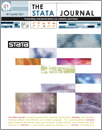Home  >>  Archives  >>  Volume 2 Number 3  >>  st0018

#### The Stata JournalVolume 2 Number 3: pp. 253-266

Subscribe to the Stata Journal## The robust variance estimator for two–stage models

 James W. Hardin Texas A&M University
Abstract.   This article discusses estimates of variance for two-stage models. We present the sandwich estimate of variance as an alternative to the Murphy–Topel estimate. The sandwich estimator has a simple formula that is similar to the formula for the Murphy–Topel estimator, and the two estimators are asymptotically equal when the assumed model distributions are true. The advantages of the sandwich estimate of variance are that it may be calculated for the complete parameter vector, and that it requires estimating equations instead of fully specified log likelihoods.

View all articles by this author: James W. Hardin

View all articles with these keywords: robust variance estimator, Murphy–Topel estimator, two-stage estimation, estimating equation